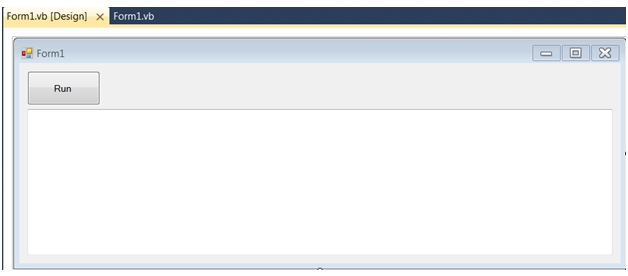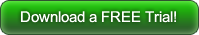## XLeratorDLL vs Worksheetfunction: XIRR

Aug 21

Written by: Charles Flock
8/21/2016 3:29 PMIn this article we take a detailed look at the XLeratorDLL function XIRR and Worksheetfunction.XIRR. You might be surprised about some of the quirks in Worksheetfunction.XIRR
I have the following statements at the top of my code.
Option Explicit On
Option Strict On

Imports System.Runtime.InteropServices
Imports System.Text
Imports Excel = Microsoft.Office.Interop.Excel
Imports wct = XLeratorDLL_financial.XLeratorDLL_financial
Then I create a very simple form which consists of a single button and a text box where the results are displayed.XIRR calculates the Internal Rate of Return (IRR) for a series of irregular cash flows. I am going to create one Class for examples using the XLDLL functions and another Class for the Excel functions. To calculate the XIRR we need a series of cash flows, the dates associated with those cash flows and a guess value. We will display the results in the text box on our form. Here's the code for XLDLL.
Public Class test_XLDLL
Public Shared Sub testXIRR()
Dim cf As Double()
Dim cfDate As Date()
Dim result As Double

cf = New Double() {-100000, -50000, -2500, 12500, 37500, 75000, 90000}
cfDate = New Date() {#11/30/2011#, #3/15/2012#, #7/18/2012#, #11/30/2012#, #1/23/2013#, #4/30/2013#, #2/6/2014#}

result = wct.XIRR(cf, cfDate, wct.NULL_DOUBLE)

Form1.TextBox1.AppendText("XLDLL XIRR: " & result.ToString("F8") & vbCrLf)

End Sub
End Class
Here's the code for the Excel version.
Public Class test_XL
Public Shared Sub testXIRR()
Dim XL As New Excel.Application
Dim cf As Double()
Dim cfdate As Date()
Dim result As Double

cf = New Double() {-100000, -50000, -2500, 12500, 37500, 75000, 90000}
cfdate = New Date() {#11/30/2011#, #3/15/2012#, #7/18/2012#, #11/30/2012#, #1/23/2013#, #4/30/2013#, #2/6/2014#}

result = XL.WorksheetFunction.Xirr(cf, cfdate)

Form1.TextBox1.AppendText("Excel XIRR: " & result.ToString("F8") & vbCrLf)

XL.Quit()

End Sub
End Class
To test the 2 functions I add the following code to Button1_Click
Public Class Form1

Private Sub Button1_Click(sender As System.Object, e As System.EventArgs) Handles Run.Click
TextBox1.Clear()
test_XL.testXIRR()
test_XLDLL.testXIRR()
End Sub

End Class
When I run this code, I get the following results.
As you can see, both functions return the same result. Using Worksheetfunction.XIRR requires a little more code to instantiate the Excel.Application (yes, using Worksheetfunction.XIRR does actually start an Excel process) and you need to makes sure that you quit the application, but the code is essentially the same. I am not going to make any arguments about the technical advantages of using a .NET DLL vs. COM, because Worksheetfunction.XIRR actually has other problems that I think are far more serious.
Speed
Let's change our code to look at the speed issue. Many of our clients using the XIRR function have hundreds of thousands to several millions of cash flows which generally are distributed across many thousands of accounts and, at the very least, they want to calculate the internal rate of return for each account. If we simply took our existing example and put it in a loop and kept track of the time in the loop we could get a pretty good feel for what the difference in speed might look like.
Let's implement the following code in test_XLDLL.
Public Shared Sub testXIRR()
Dim cf As Double()
Dim cfDate As Date()
Dim result As Double

cf = New Double() {-100000, -50000, -2500, 12500, 37500, 75000, 90000}
cfDate = New Date() {#11/30/2011#, #3/15/2012#, #7/18/2012#, #11/30/2012#, #1/23/2013#, #4/30/2013#, #2/6/2014#}

Dim time_start As Date
Dim time_end As Date

time_start = Now
For i = 1 To 50000
result = wct.XIRR(cf, cfDate, wct.NULL_DOUBLE)
Next
time_end = Now

Form1.TextBox1.AppendText("XLDLL XIRR elapsed time: " & (time_end - time_start).TotalMilliseconds.ToString("F2") & " millisecond" & vbCrLf)

End Sub
And, in test_XL.
Public Shared Sub testXIRR()
Dim XL As New Excel.Application
Dim cf As Double()
Dim cfdate As Date()
Dim result As Double

cf = New Double() {-100000, -50000, -2500, 12500, 37500, 75000, 90000}
cfdate = New Date() {#11/30/2011#, #3/15/2012#, #7/18/2012#, #11/30/2012#, #1/23/2013#, #4/30/2013#, #2/6/2014#}

Dim time_start As Date
Dim time_end As Date

time_start = Now
For i As Integer = 1 To 50000
result = XL.WorksheetFunction.Xirr(cf, cfdate)
Next
time_end = Now

Form1.TextBox1.AppendText("Excel XIRR elapsed time: " & (time_end - time_start).TotalMilliseconds.ToString("F2") & " milliseconds" & vbCrLf)

XL.Quit()

End Sub
This produces the following result.
This code is calculating the same XIRR 50,000 times using the same seven cash flows. The XLDLL calculation runs approximately 50 times faster than the Excel calculation. It’s pretty clear that if your volume is large (whatever you consider large to be), then XLeratorDLL is the way to go.
Reliability
Let's look at the reliability issue. The first issue is a problem that we first identified in Excel in December of 2008, which is that the order of input may affect the results.
Let's make the following changes to our XLDLL code:
Public Shared Sub testXIRR()
Dim cf As Double()
Dim cfDate As Date()
Dim result As Double

cf = New Double() {-88.544, 21.343, 60.169, -41.718, 79.444, 13.106, -95.013, 32.164, -21.048, 19.815, -34.443, -20.953, -83.28, 2.112, 87.678, 11.139}
cfDate = New Date() {#11/16/2005#, #5/21/2005#, #3/18/2002#, #11/20/2002#, #5/15/2006#, #7/10/2008#, #7/19/2011#, #9/15/2013#, #2/2/2014#, #3/24/2016#, #10/10/2013#, #8/27/2014#, #4/22/2011#, #12/17/2009#, #2/2/2007#, #6/6/2010#}

result = wct.XIRR(cf, cfDate, wct.NULL_DOUBLE)

Form1.TextBox1.AppendText("XLDLL XIRR (unsorted): " & result.ToString("F8") & vbCrLf)

Array.Sort(cfDate, cf)

result = wct.XIRR(cf, cfDate, wct.NULL_DOUBLE)

Form1.TextBox1.AppendText("XLDLL XIRR (sorted): " & result.ToString("F8") & vbCrLf)

End Sub
The code takes the input cash flow and cash flows dates, which are in no particular order and calls XIRR. It then sorts that date and amounts in date order and calls XIRR. The two results are appended to the text box.
Here is the code for the Excel version.
Public Shared Sub testXIRR()
Dim XL As New Excel.Application
Dim cf As Double()
Dim cfdate As Date()
Dim result As Double

cf = New Double() {-88.544, 21.343, 60.169, -41.718, 79.444, 13.106, -95.013, 32.164, -21.048, 19.815, -34.443, -20.953, -83.28, 2.112, 87.678, 11.139}
cfdate = New Date() {#11/16/2005#, #5/21/2005#, #3/18/2002#, #11/20/2002#, #5/15/2006#, #7/10/2008#, #7/19/2011#, #9/15/2013#, #2/2/2014#, #3/24/2016#, #10/10/2013#, #8/27/2014#, #4/22/2011#, #12/17/2009#, #2/2/2007#, #6/6/2010#}

result = XL.WorksheetFunction.Xirr(cf, cfdate)

Form1.TextBox1.AppendText("Excel XIRR (unsorted): " & result.ToString("F8") & vbCrLf)

Array.Sort(cfdate, cf)

result = XL.WorksheetFunction.Xirr(cf, cfdate)

Form1.TextBox1.AppendText("Excel XIRR (sorted): " & result.ToString("F8") & vbCrLf)

XL.Quit()

End Sub
This produces the following result.
As you can see, Worksheetfunction.XIRR produces 2 different answers depending on the order of the input. Which one are you reporting to management or your customers?
Here’s another example of the unreliability of Worksheetfunction.XIRR. Make the following changes to the code for XLDLL.
Public Shared Sub testXIRR()
Dim cf As Double()
Dim cfDate As Date()
Dim result As Double

cf = New Double() {3000, -55000, 15000, 60000}
cfDate = New Date() {#1/15/2010#, #1/15/2011#, #1/15/2012#, #1/14/2013#}

result = wct.XIRR(cf, cfDate, wct.NULL_DOUBLE)
Form1.TextBox1.AppendText("XLDLL XIRR: " & result.ToString("F8") & vbCrLf)

End Sub
Make these changes for the Excel code.
Public Shared Sub testXIRR()
Dim XL As New Excel.Application
Dim cf As Double()
Dim cfdate As Date()
Dim result As Double

cf = New Double() {3000, -55000, 15000, 60000}
cfdate = New Date() {#1/15/2010#, #1/15/2011#, #1/15/2012#, #1/14/2013#}

result = XL.WorksheetFunction.Xirr(cf, cfdate)
Form1.TextBox1.AppendText("Excel XIRR: " & result.ToString("F8") & vbCrLf)

XL.Quit()
End Sub
When we run the code we get the following result.
The Excel result returns zero. How can that possibly be? In the real world pretty much the only way to return a value of zero is for the sum of the cash flows to be zero, which is certainly not the case here. Again, this is a bug in the Excel calculation which we have documented in several other blogs.
One further point to make here is that an XIRR of zero is not an indication that Excel could not find a solution. First of all, there is a solution as you can see from the XLDLL result. Second the documentation for the Excel function states: If XIRR can't find a result that works after 100 tries, the #NUM! error value is returned.
Let's look at an example for which there is no solution and see how Excel and XLDLL handle it. Make the following changes to the XLDLL code.
Public Shared Sub testXIRR()
Dim cf As Double()
Dim cfDate As Date()
Dim result As Double

cf = New Double() {-200000, -20000, 74000, -1000, -30000, -999.6}
cfDate = New Date() {#6/11/2016#, #6/17/2016#, #6/22/2016#, #6/27/2016#, #6/28/2016#, #6/29/2016#}

result = wct.XIRR(cf, cfDate, wct.NULL_DOUBLE)

Form1.TextBox1.AppendText("XLDLL XIRR: " & result.ToString("F8") & vbCrLf)

End Sub
And make the following changes to the Excel code.
Public Shared Sub testXIRR()
Dim XL As New Excel.Application
Dim cf As Double()
Dim cfdate As Date()
Dim result As Double

cf = New Double() {-200000, -20000, 74000, -1000, -30000, -999.6}
cfdate = New Date() {#6/11/2016#, #6/17/2016#, #6/22/2016#, #6/27/2016#, #6/28/2016#, #6/29/2016#}

Try
result = XL.WorksheetFunction.Xirr(cf, cfdate)
Catch
result = Double.NaN
End Try

Form1.TextBox1.AppendText("Excel XIRR: " & result.ToString("F8") & vbCrLf)

XL.Quit()

End Sub
Notice that I added Try…Catch logic to the Excel code to trap the error. There's no need to do this for the XLDLL code as the function anticipates (correctly) that there might be conditions under which a solution is not obtainable and returns a meaningful value rather than an error.
It seems pretty clear that the Excel documentation is accurate and the #NUM! error value is returned if the function cannot find a solution (though why it thinks this is an error causes me to scratch my head; it's not like every series of cash flows has an internal rate of return).
One final example. Change your XLDLL code to the following.
Public Shared Sub testXIRR()
Dim cf As Double()
Dim cfDate As Date()
Dim result As Double

cf = New Double() {0, -5940.37, -4833.84, 11259.59}
cfDate = New Date() {#4/11/2016#, #9/15/2017#, #6/9/2017#, #3/31/2018#}
cf(0) = -wct.XNPV(0.07604, cf, cfDate)

result = wct.XIRR(cf, cfDate, wct.NULL_DOUBLE)
Form1.TextBox1.AppendText("XLDLL XIRR: " & result.ToString("F8") & vbCrLf)

End Sub
What we did was create a series of cash flows, with zero as the initial cash flow and then calculated the initial cash flow using the XNPV function and a discount rate of 0.07604. Since XIRR is calculating the value for discount rate such that the XNPV is approximately zero we would expect to get the input discount rate, 0.07604, returned.
Here's the Excel code.
Public Shared Sub testXIRR()
Dim XL As New Excel.Application
Dim cf As Double()
Dim cfdate As Date()
Dim result As Double

cf = New Double() {0, -5940.37, -4833.84, 11259.59}
cfdate = New Date() {#4/11/2016#, #9/15/2017#, #6/9/2017#, #3/31/2018#}

cf(0) = -wct.XNPV(0.07604, cf, cfdate)

Try
result = XL.WorksheetFunction.Xirr(cf, cfdate)
Catch
result = Double.NaN
End Try

Form1.TextBox1.AppendText("Excel XIRR: " & result.ToString("F8") & vbCrLf)

XL.Quit()
End Sub
This produces the following result.
As you can see, XLDLL returns the expected result, while the Excel implementation does not.
Clearly the XLeratorDLL/financial XIRR function offers significant benefits just in terms of functionality that you should seriously consider using it instead of WorksheetFunction.XIRR.
You can read more about XLeratorDLL/financial here. If you have any questions or if there is anything you would like to see added to the library, feel free to contact us at support@westclintech.com.Tags:
Categories:
Location: BlogsThe WestClinTech Blog

## Search Blogs

 KeywordsPhrase

## Blog Archives

Archive
 < July 2021 >
SunMonTueWedThuFriSat
27282930123
45678910
11121314151617
18192021222324
25262728293031
1234567
Monthly
Go

### SupportCopyright 2008-2021 Westclintech LLC         Privacy Policy        Terms of Service